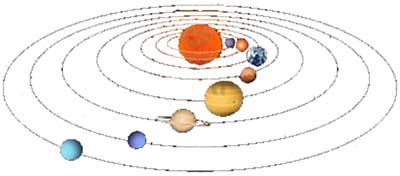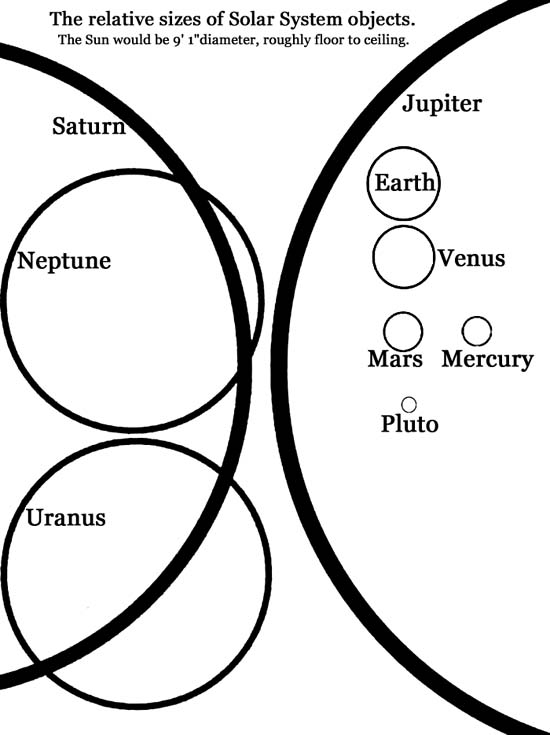Steve & Debbie Russell's Telescope Clinic

Solar System Scale Model

An attempt to describe a “manageable” scale model of the Solar System.

For this scale, we will start with the diameter of Earth to be 1”.
The Sun would then be 9’1” in diameter (roughly floor to ceiling).
Earth would be 976’ from the Sun, roughly the height of the Eiffel Tower.
Mercury would be 0.4” diameter and 378’ from the Sun.
Venus would be 0.95” diameter and 705.5’ from the Sun.
Mars would be 0.5” diameter and 1487’ from the Sun, about a quarter of a mile.
Jupiter would be 11.2” diameter and 5078’ from the Sun, almost 1 mile.
Saturn would be 9.1” diameter and 9312’ from the Sun, about 1-3/4 miles.
Uranus would be 3.7” diameter and 18,781 ‘ from the Sun, about 3.6 miles.
Neptune would be 3.6” diameter and 29, 366’ from the Sun, about 5.6 miles.
Pluto would be 0.2” diameter and 38,587’ from the Sun, about 7.3 miles.

At this scale, the speed of light would be reduced to 2’ per second!

To go one step farther, we can fit this model on one 8-1/2 x 11” piece of paper.
Use graph paper with 1/4” squares drawn on it and let each 1/4” represent 1000 feet.
The Sun, which represents far more than 99% of the Solar System’s mass, would be 0.0021” in diameter (half as thick as a piece of paper).
Pluto would be 9.5” from the Sun, but Mercury, Venus, and Earth are all less than 1/4” from the Sun!

This drawing is very much not to scale!Below is a graphic showing the relative sizes of the planets; zoom (ctrl- or ctrl+) to make Earth 1" in diameter.